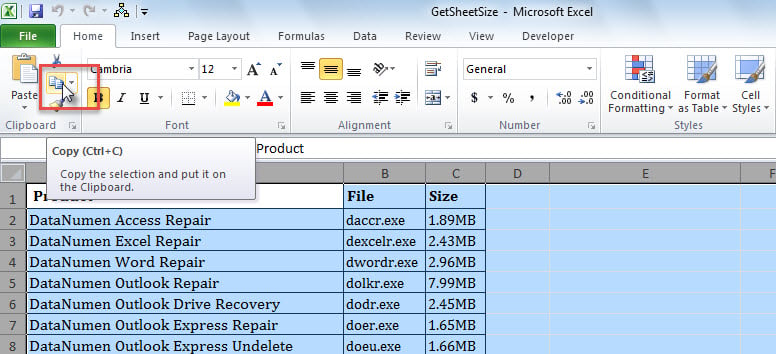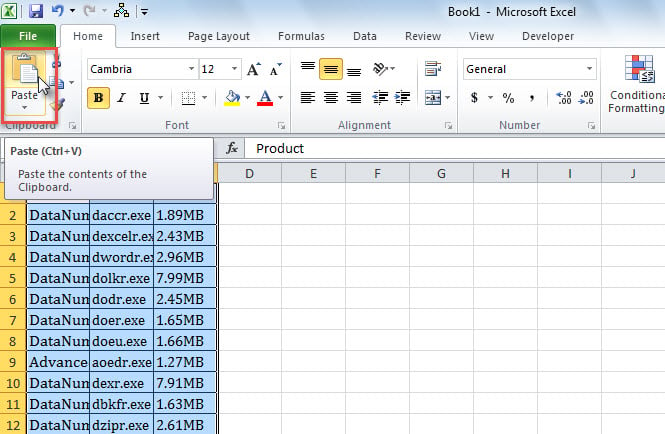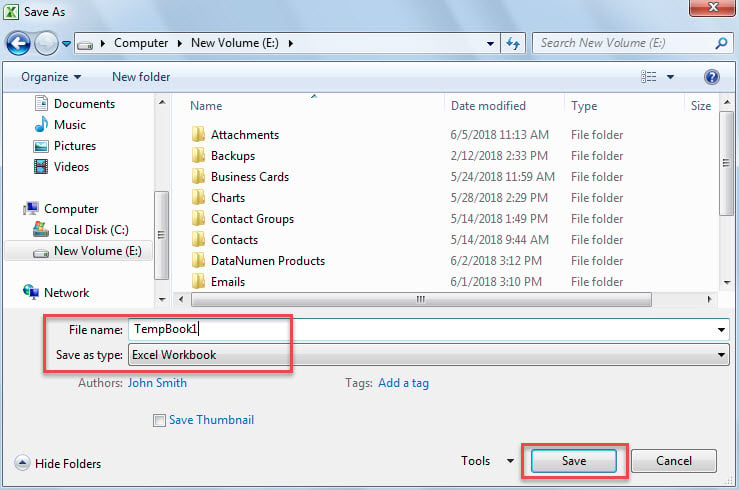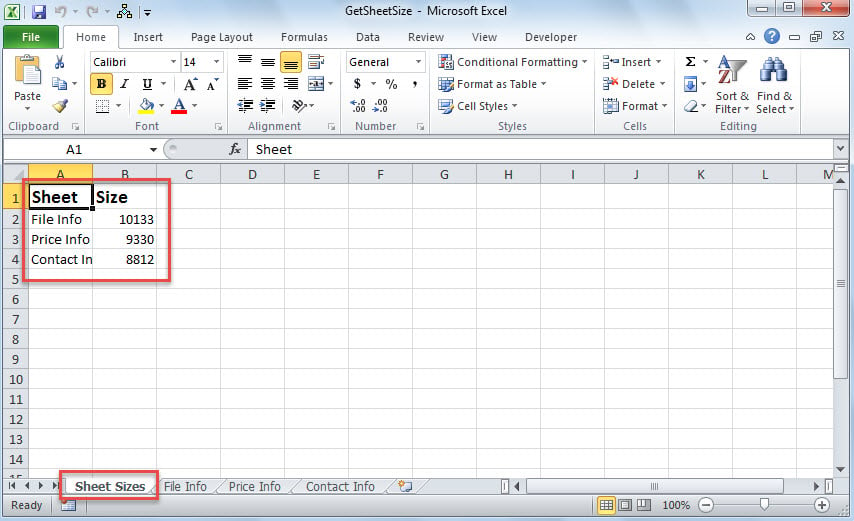# 2 Quick Ways to Get the Data Size of Each Worksheet in an Excel Workbook

If you want to quickly get the data size of each worksheet in an Excel workbook, you can refer to this article. Here we will share 2 handy methods with you.

At times, when dealing with an Excel workbook which consists of a great amount of worksheets, you may want to get the data size of each worksheet. However, there is not a native feature for this requirement. So, you need to use some workarounds, such as the following Method 1. Or you can use the much smarter Method 2 to get all worksheets’ data sizes in bulk.

## Method 1: Get Worksheet Data Size One by One Manually

1. At first, open the specific Excel workbook.
2. Then, copy the contents of an Excel worksheet.3. Next, create a new workbook and paste the copied worksheet into Sheet 1.4. Later, save the new workbook to a Windows folder.5. Lastly, you can check the new workbook’s data size, equal to the worksheet’s data size, in Windows Explorer, as shown in the following screenshot.6. Now, you can use this way to get the data sizes of other worksheets one by one.

## Method 2: Batch Get the Data Sizes of All Worksheets via VBA

1. To begin with, open the Excel VBA editor according to “How to Run VBA Code in Your Excel“.
2. Then, put the following code into a project or module.
```Sub GetEachWorksheetSize()
Dim strTargetSheetName As String
Dim strTempWorkbook As String
Dim objTargetWorksheet As Worksheet
Dim objWorksheet As Worksheet
Dim objRange As Range
Dim i As Long
Dim nLastEmptyRow As Integer

strTargetSheetName = "Sheet Sizes"
strTempWorkbook = ThisWorkbook.Path & "\Temp Workbook.xls"

.Name = strTargetSheetName
.Cells(1, 1) = "Sheet"
.Cells(1, 1).Font.Size = 14
.Cells(1, 1).Font.Bold = True
.Cells(1, 2) = "Size"
.Cells(1, 2).Font.Size = 14
.Cells(1, 2).Font.Bold = True
End With

Set objTargetWorksheet = Application.Worksheets(strTargetSheetName)

For Each objWorksheet In Application.ActiveWorkbook.Worksheets
If objWorksheet.Name <> strTargetSheetName Then
objWorksheet.Copy

Application.ActiveWorkbook.SaveAs strTempWorkbook
Application.ActiveWorkbook.Close SaveChanges:=False

nLastEmptyRow = objTargetWorksheet.Range("A" & objTargetWorksheet.Rows.Count).End(xlUp).Row + 1

With objTargetWorksheet
.Cells(nLastEmptyRow, 1) = objWorksheet.Name
.Cells(nLastEmptyRow, 2) = FileLen(strTempWorkbook)
End With

Kill strTempWorkbook
End If
Next
End Sub```1. After that, press “F5” to run this macro right away.
2. Eventually, after macro finishes, back to the workbook.
3. You’ll see a new worksheet at the very beginning, which contains the size of each worksheet, like the following image.## Comparison

 Advantages Disadvantages Method 1 Easy to operate Too troublesome if there are too many worksheets in the current workbook Method 2 Easy and convenient no matter how many worksheets exist in the workbook Perhaps the above VBA code is a bit incomprehensible for newbies

## Fix Exasperating PST Issues

Users can encounter all kinds of disturbing troubles in MS Excel, ranging from unexpected shutdown or restart to severe xlsx corruption. Unquestionably, the most knotty problem is damaged Excel file in that it cannot be resolved easily. It demands you to apply an external Excel fix tool, like DataNumen Excel Repair.

## Author Introduction:

Shirley Zhang is a data recovery expert in DataNumen, Inc., which is the world leader in data recovery technologies, including corrupt SQL Server and outlook repair software products. For more information visit www.datanumen.com